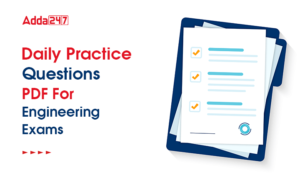Engineering Jobs   »   QUIZ : CIVIL ENGINEERING (23-09-2020)

# QUIZ : CIVIL ENGINEERING (23-09-2020)

Quiz: Civil Engineering
Exam: Assam -Psc
Topic: Strength of Material
Each question carries 1 mark
Negative marking: No negative marking
Time: 10 Minutes

Q1. In the creep test, the following type of stress is applied to the specimen
(a) uniaxial compression
(b) uniaxial tension
(c) biaxial compression or tension
(d) alternating stress

Q2. A free bar of length ℓ is uniformly heated from 0°C a temperature t°C, α is the coefficient of linear expansion and E is the modulus of elasticity. The stress in the bar is
(a) α t E
(b) α t E/2
(c) zero
(d) None of these

Q3. If Poisson’s ratio for a material is 0.5, then the elastic modulus for the material is
(a) three times its shear modulus
(b) four times its shear modulus
(c) equal to its shear modulus
(d) indeterminate

Q4. The Poisson’s ratio of a material which has Young’s modulus of 120 GPa, and shear modulus of 50 GPa, is
(a) 0.1
(b) 0.2
(c) 0.3
(d) 0.4

Q5. Solid uniform metal bar of diameter D and length L is hanging vertically from its upper end. The elongation of the bar due to self weight is
(a) Proportional to L and inversely proportional to D²
(b) Proportional to L² and inversely proportional to D²
(c) Proportional to L but independent of D
(d) Proportional to L² but independent of D

Q6. The stress below which a material has a high probability of not failing under reversal of stress is known as
(a) tolerance limit
(b) elastic limit
(c) proportional limit
(d) endurance limit

Q7. In stress-strain curve, the area upto elastic limit stress indicates which mechanical property?
(a) Ductility
(b) Strength
(c) Resilience
(d) None of these

Q8. True stress σ is related with conventional stress σ_0 as
(a) σ/σ_0 =(1+ϵ)^2
(b) 〖σ/σ〗_0=1/(1+ϵ)^2
(c) 〖σ/σ〗_0=1/(1+ϵ)
(d) σ/σ_0 =1+ϵ

Q9. The radius of Mohr’s circle of stress of a strained element is 20 mm and minor principal tensile stress is 10 N/mm². The major principal stress is
(a) 30 N/mm²
(b) 50 N/mm²
(c) 60 N/mm²
(d) 100 N/mm²

Q10. The principal stresses σ_1,σ_2 and σ_3 at a point respectively are 80 MPa, 30 MPa and – 40 Mpa. The maximum shear stress is
(a) 25 MPa
(b) 35 MPa
(c) 55 MPa
(d) 60 MPa

SOLUTION

S1. Ans.(b)
Sol. In creep test uniaxial tension is applied to the specimen.

S2. Ans.(c)
Sol. The bar is free to move then there is no stress occur in the bar due to thermal expansion.

S3. Ans.(a)
Sol. E=2G(1+μ)
E=2G(1+0.5)
▭(E=3G)

S4. Ans.(b)
Sol. E=120 GPa
G=50 GPa

E=2G(1+μ)
120=2×50(1+μ )

1+μ=120/100
▭(μ=0.2)

S5. Ans.(d)
Sol. Elongation of bar due to self weight
▭(∆L=(YL^2)/2E)
▭(∆L α L^2 )

S6. Ans.(d)

S7. Ans.(c)

S8. Ans.(c)
Sol. ▭(σ/σ_0 =1/(1+ϵ))
σ- true stress
σ_0-conventional stress
ϵ=strain

S9. Ans.(b)
Sol. Radius of mohr circle =(σmax-σmin)/2
20=(σmax-10)/2
▭(σ_max=50N\/mm^2 )

S10. Ans.(d)
Sol. σ_1=80 MPa
σ_2=30 MPa
σ_3=-40 MPa
Maximum shear stress =(σ_1-σ_3)/2
=(80-(-40))/2
=60MPa

Sharing is caring!

•GATE Result 2023 Out, Download Result, C...
•GATE 2023 Admit Card Out, Download Link ...
•Daily Practice Questions PDF For Enginee...
•UKPSC JE Exam Pattern 2023, Check Detail...
•ICF Chennai Apprentice Recruitment 2023 ...
•CDAC Recruitment 2023, Apply Online for ...
•JSSC JE Previous Year Papers and Cutoff,...
•BHEL Recruitment 2023, Notification, App...